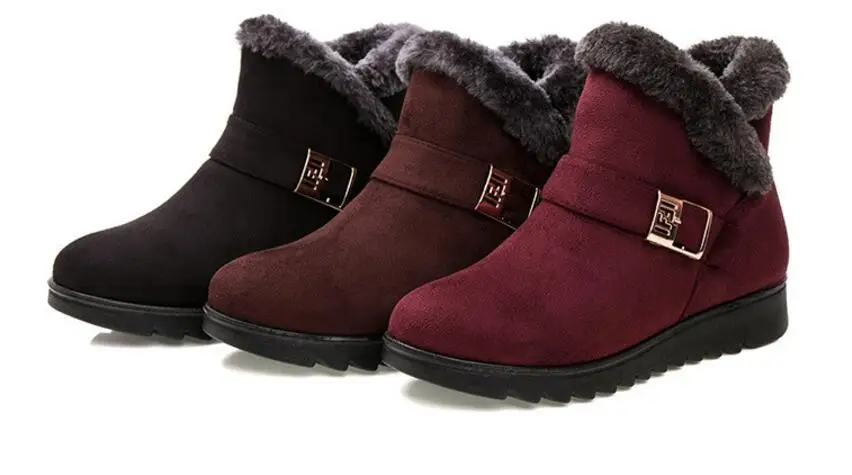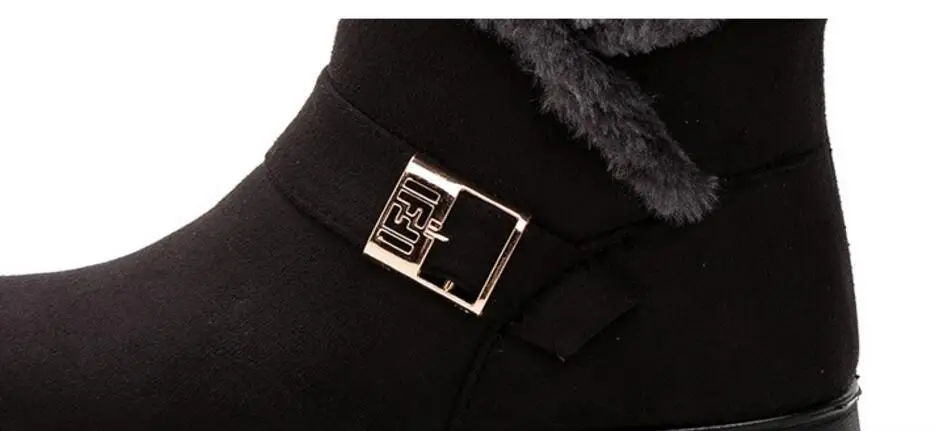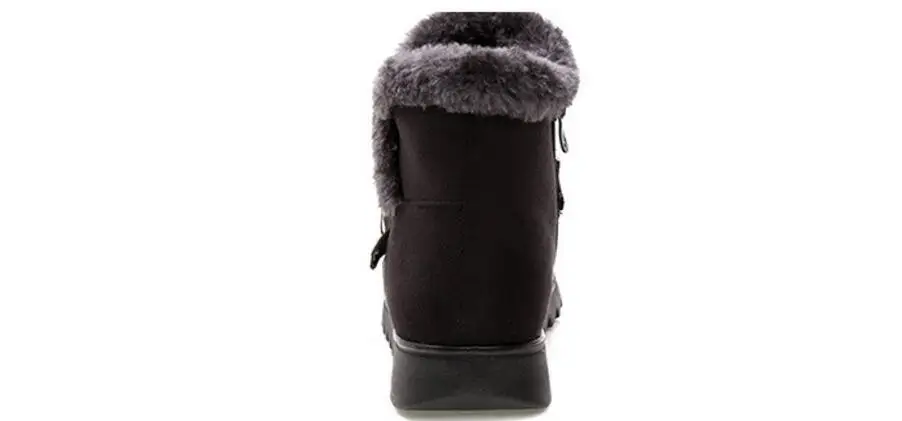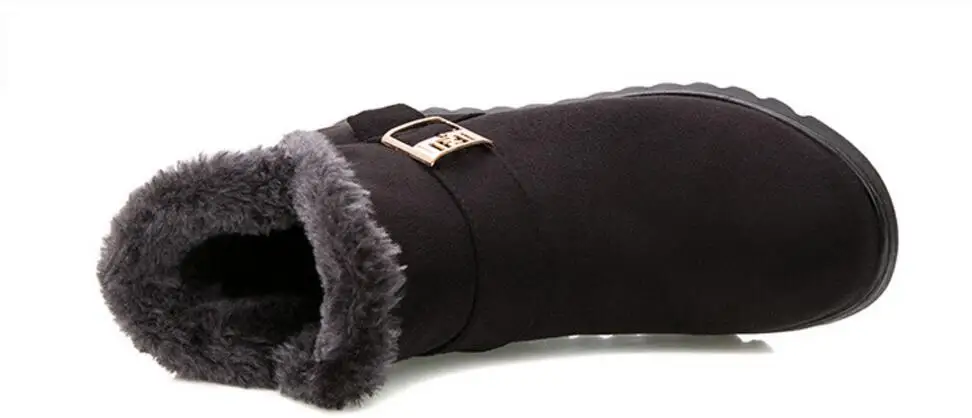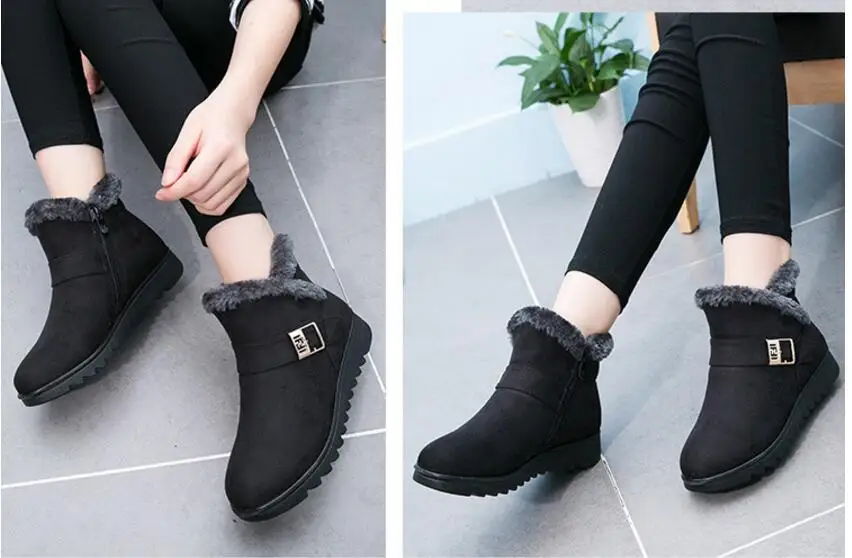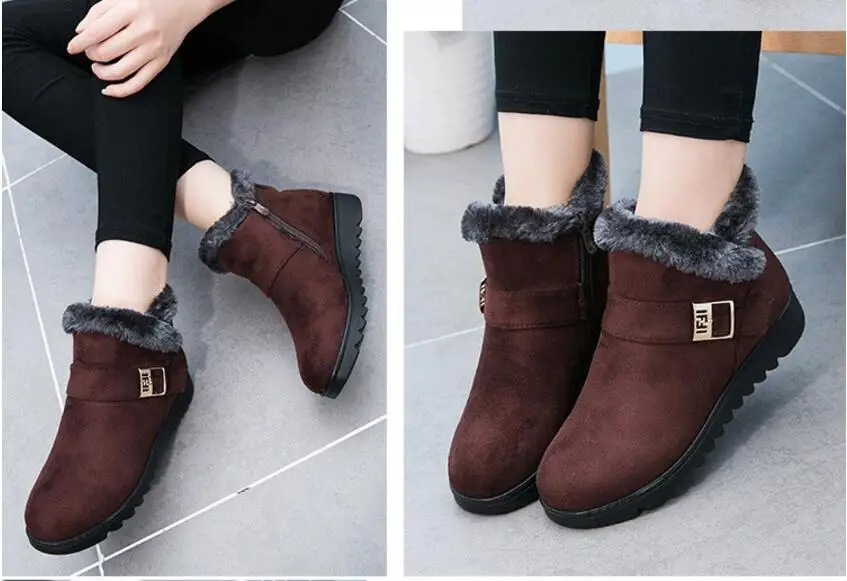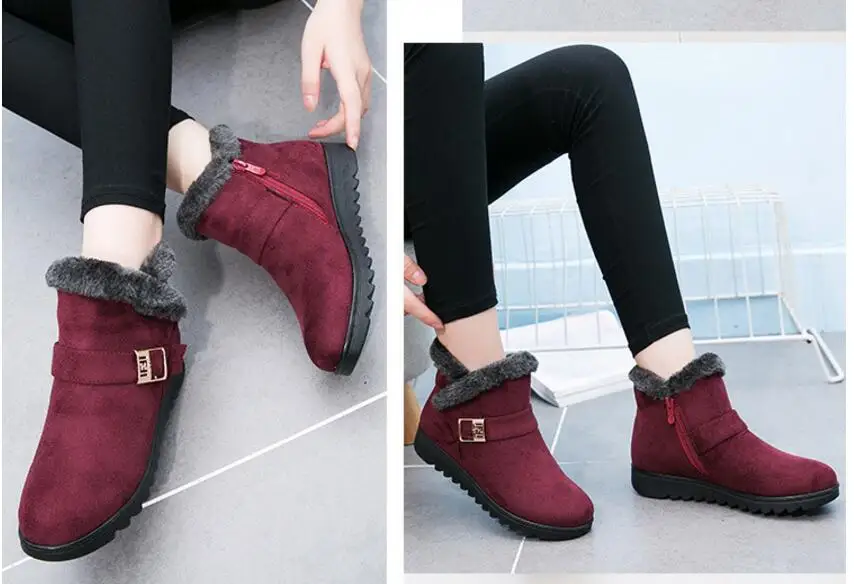# Snow Boots – Merlot – 3 Colors

• ### Easy Returns

Return policy that lets you shop at ease
• ### Always Authentic

We only sell 100% authentic products
• ### Secure Shopping

18.60

 Color BlackBrownWine Red Shoe Size 1010.5566.57.58.599.5Clear
SKU: 33018575931 Categories: ,

## Snow Boots – Trendy

Material: Flock, Rubber

Color: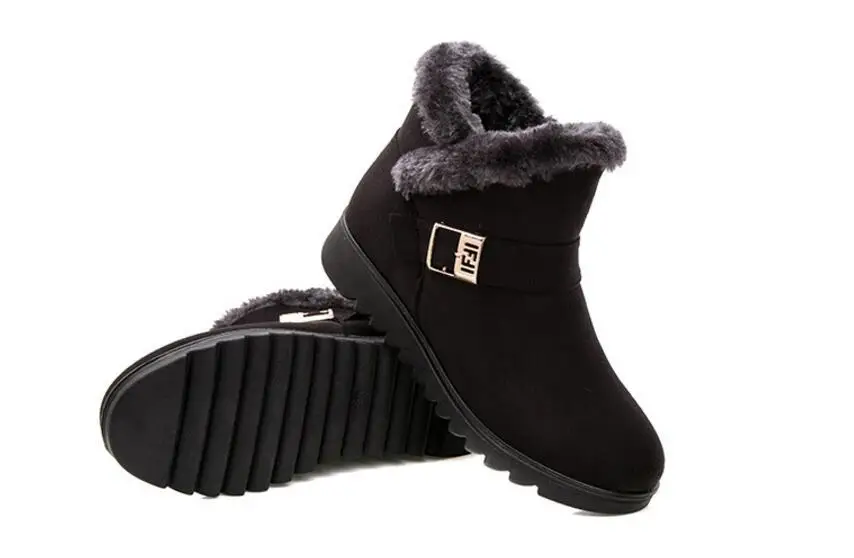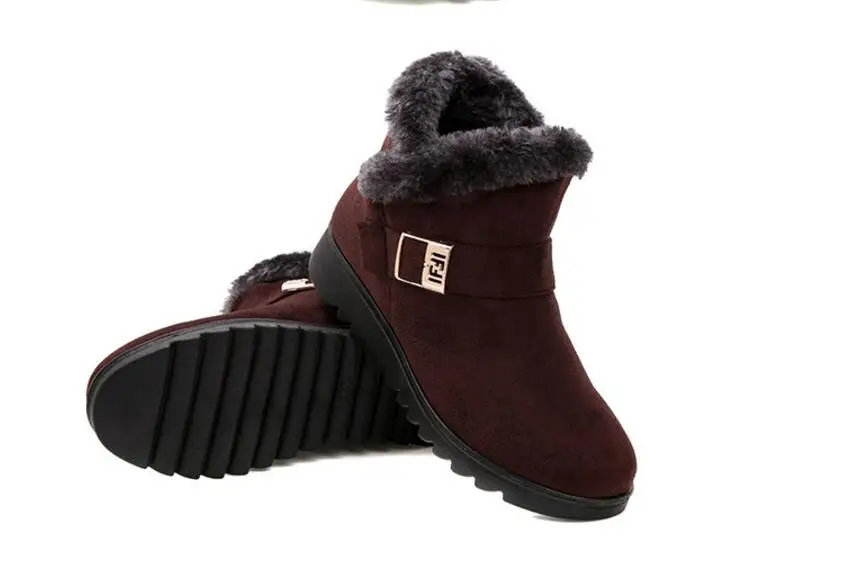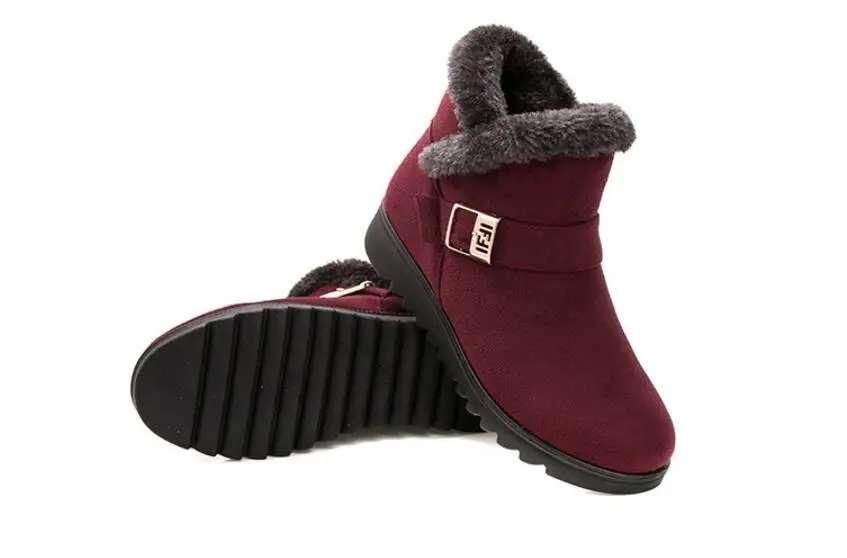SIZE INFO

Note:

• Due to the different monitor and light effect, the actual color of the item might be slightly different from the color showed on the pictures. Thank you!
• Please allow 1-3cm measuring error due to manual measurement. 1 inch = 2.54 cm, 1 cm = 0.39 inch.

34 = 22cm =         4 US

35 = 22.5cm =      5 US

36 = 23cm =         6 US

37 = 23.5cm =   6.5 US

38 = 24cm =      7.5 US

39 = 24.5cm =  8.5 US

40 = 25cm =        9 US

41 = 25.5cm =   9.5 US

42 = 26cm =       10 US

43 = 26.5cm = 10.5 US

44 = 27cm =        11 US

45 = 27.5cm =  11.5 US

46 = 28cm =       12 US

47 = 28.5cm = 12.5 US

48 = 29cm =       13 US